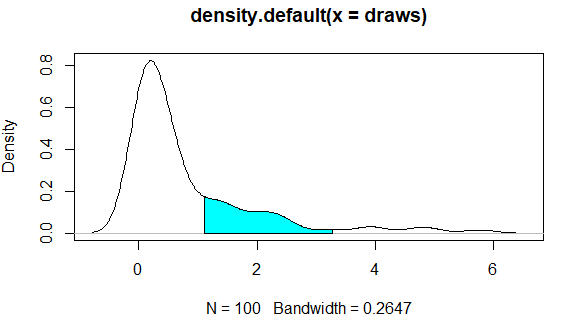2 views

I frequently use kernel density plots to illustrate distributions. These are easy and fast to create in R like so:

set.seed(1)

draws <- rnorm(100)^2

dens <- density(draws)

plot(dens)

#or in one line like this: plot(density(rnorm(100)^2))

Which gives me this nice little PDF:I'd like to shade the area under the PDF from the 75th to 95th percentiles. It's easy to calculate the points using the quantile function:

q75 <- quantile(draws, .75)

q95 <- quantile(draws, .95)

But how do I shade the the area between q75 and q95?

by

To shade the plot you can use the polygon function that draws the polygons whose vertices are given in x and y.

set.seed(1)

draws <- rnorm(100)^2

dens <- density(draws)

plot(dens)

q75 <- quantile(draws, .75)

q95 <- quantile(draws, .95)

x1 <- min(which(dens\$x >= q75))

x2 <- max(which(dens\$x <  q95))

with(dens, polygon(x=c(x[c(x1,x1:x2,x2)]), y= c(0, y[x1:x2], 0), col="cyan"))

Output: